# Probability definition

## what is probability

Probability is the measure of the likeliness that an event will occur. Probability is quantified as a number between 0 and 1 (where 0 indicates impossibility and 1 indicates certainty)

## Why we need Probability and what is the use of it?

It is widely used in the study of Mathematics, Statistics, Gambling, Physical sciences, Biological sciences, Weather forecasting, Finance etc. to draw conclusions. Insurance companies uses this to decide on financial policies

## Terms related to Probability

### Randomness or Random experiments

• When we perform experiments in science and engineering repeatedly under very nearly identical conditions, we almost get the same result.
• Such experiments are called deterministic experiments.
• There also exists experiments When next outcome of the experiment cannot be determined  even they are performed under nearly identical conditions then we say it is a random experiment
• e.g. Consider the dice. When we throw the dice, we cannot determine what number will come Since we cannot predict the next outcome, we may say it is a random experiment
• Probability theory is concerned with such random phenomena or random experiments

### Trial

A trial is an action which results in one or several outcomes, for example each toss of the coin and each throw of the die are called trials

#### Independent Trial

Successive trials of some random event for example tosses of a coin,throws of a die are said to be independent if the outcome of any one trial does not impact the outcomes of any others.

### Sample space

• It is a set of all possible outcomes of an random experiment which we defined earliar
e.g. when we coin is tossed, the possible outcome are Head and Tail.So sample space is Head and tail
• It is generally denoted by letter S
• The sample space can be either be finite or infinite.
• Example for infinite Sample space would be an experiment where we are  tossing the coin    until Head comes up.
Sample space { (H), (TH),(TTH), (TTTH)..}
• We can use Tree diagram to find out the sample space. Permutation and combination studied in earliar chapter also  will be used extensively to find the sample space

### Elementary event

Each outcome of the random experiment is known as elementary events

### Event

• An event is a possible outcome of the Experiment. Any subset E of the sample space S is called an event.
• Example of events
1. Roll a die: the outcome is even {2, 4, 6}.
2. Flip a coin twice and the two results are different:
{(H, T),(T, H)}.

### Different type of events

 Elementary or simple event If it contains the single elements of the sample space Compound event If it contains more than single elements of the sample space Impossible event If it can never occur. Sure or certain event An event which is sure to occur

## Algebra of events operations

We know the event are sets and they are subset of Sample space. So normal set operations can be done over them also
Let A and B are two events of the Sample Space S
 Union: (A ∪ B) an outcome is in A ∪ B if it is either in A or in B.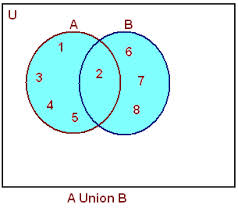Intersection: (A ∩ B), AB: an outcome is in AB if it is in both A and B.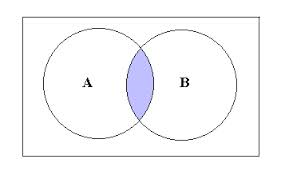Difference (A -B)  , the event of occurrence of A but not B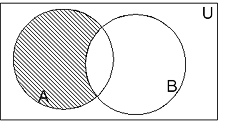Difference (B -A)  , the event of occurrence of B but not A AC  : The event of non-occurrence of the event A.It is also called the complementary event of A

## Some More Important Definition on Probability

### Equally Likely Outcomes

The outcome of the random experiments are called equally likely outcomes. If all of them are equal preferences.

### Exhaustive Outcomes

The outcome of the random experiments are called exhaustive  outcomes. If they cover all the possible outcomes of the experiments

## Theoretical probability definition or Classical Probability

The theoretical probability or the classical probability of the event A in a random experiment having exhaustive, equal likely outcome is defined as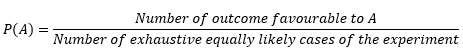1. Let Number of exhaustive equally likely cases of the experiment =n
Number of outcome favorable to A=m
Then
$P( A) = \frac {m}{n}$
Also you may have observed that
$0 \leq m \leq n$
Or
$0 \leq \frac {m}{n} \leq 1$
2. The event $A^c$ representing ‘not A’, is called the complement of the event A. We also say that $A^c$ and $A$ are complementary events.
Now
$P(A^c) = \frac {n-m}{n} = 1 - \frac {m}{n} = 1- P(A)$
Or
$P(A) +P(A^c)=1$
3. The probability of an event (U) which is impossible to occur is 0. Such an event is called an impossible event
$P(U)=0$
4. The probability of an event ( X) which is sure (or certain) to occur is 1. Such an event is called a sure event or a certain event
$P(X)=1$
/li>
5. Probability of any event can be as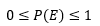6. Odds in favor of the happening of the event is defined as ratio of P(A) and P(Ac/sup>)
Let it be    m: n
Then $\frac {P(A)} {P(A^c)}=\frac { m}{n}$
Or
$\frac {P(A)}{1- P(A)} =\frac {m}{n}$
Or
$P(A) = \frac {m}{(m + n)}$
7. Odds against the happening of the event is defined as ratio of P(Ac) and P(A)
Let it be m: n
Then
$P(A) =\frac {n}{ (m + n)}$

## How to Solve Probability Problems

1. Find out the number of elements in the sample space S of the given random experiment. It would be nice to write sample space elements also if possible
2. Find out the elements which lies in the event (A) which we are looking to find probability. Count the elements in it
3. Both the step 1 and step 2 depends on the complexity of the problem. We may have to use  Permutation and combination for arrangement questions
4.  Use classical definition of probability to find out the answer

## Related Topics

Go back to Class 12 Main Page using below links